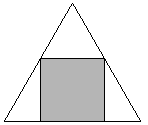# How to maximize area of a square inscribed in a equilateral triangle?

We have an equilateral triangle and want to inscribe a square, in such way that maximizes the area of the square.I sketched two possible ways, not to scale and not perfect.

Note I am not sure if the second way will really have all square corners touching the triangle sides.

The second case appears to have bigger side-lengths of the square, so bigger area. But I do not know how to determine the angles involved. How to solve this?

• @TobyMak This isn't a duplicate, since OP is asking which of two specific configurations is better. – Parcly Taxel Aug 13 '19 at 2:53
• How do you define "inscribed"? In your first sketch, one corner of the square is actually floating, not touching the triangle. I think it is safe to assume that there is only one way to inscribe a square in an equilateral triangle, and it is when one side of the square lays exactly on one side of the triangle (as in your second sketch). As a result, the problem of maximization is a non-problem. – virolino Aug 13 '19 at 11:04
• @ParclyTaxel Are they? Because that's not the question asked in the title. – Jack M Aug 13 '19 at 11:22
• @JackM It is in the consideration of the title problem that the real question has been asked. – Parcly Taxel Aug 13 '19 at 11:24
• Possible duplicate of What is the maximum area of a square inscribed in an equilateral triangle? – Jam Nov 20 '19 at 19:01Let $$a_1$$ and $$a_2$$ be the side lengths of the two squares. To determine which one is larger, we simply look at their ratio below.

With the angles in the diagram,

$$d_1=\frac{1}{2\tan 30}a_1=\frac{\sqrt{3}}{2}a_1$$ $$d_2=\frac{\sin 15}{\sin 30}a_2=\frac{1}{2\cos 15}a_2$$

Assume both equilateral triangles have unit height.

$$1=a_1+d_1=\left(1+\frac{\sqrt{3}}{2}\right)a_1=\frac{1}{2}(2+\sqrt{3})a_1$$ $$1=\sqrt{2}a_2+d_2=\left(\sqrt{2}+\frac{1}{2\cos 15}\right)a_2=\frac{1}{2}(\sqrt{2}+\sqrt{6})a_2$$

So, their ratio is

$$\frac{a_1}{a_2}= \frac{\sqrt{2}+\sqrt{6}}{2+\sqrt{3}} =\left(\frac{8+4\sqrt{3}}{7+4\sqrt{3}}\right)^{\frac{1}{2}} > 1$$

The second configuration (square has edge contact with triangle) indeed has a bigger inscribed square. If the square has unit sides, the triangle's side is $$1+\frac2{\sqrt3}$$:The symmetric first configuration may be resolved as follows. Set the unit square's bottom corner as $$(0,0)$$, so that the top corner is $$(0,\sqrt2)$$. Let the side length of the triangle be $$r$$. Then we have, by similar triangles, $$\frac{(\sqrt3/2)r-\sqrt2/2}{\sqrt2/2}=\sqrt3$$ $$r=(1+\sqrt3)\sqrt{\frac23}=2.230\dots$$ and this is greater than $$1+\frac2{\sqrt3}=2.154\dots$$, so the first configuration has a smaller inscribed square than the second.

• Maybe, you mean the second, in your the sentence before last? – dmtri Aug 18 '19 at 9:51
• @dmtri I got my words right there, checking again. – Parcly Taxel Aug 18 '19 at 9:52
• Sorry , you are right , you are talking about the sides of the triangle not the square.... – dmtri Aug 20 '19 at 18:36

Let sides-lengths of the equilateral triangle be equal to $$1$$.

Let $$x$$ be sides-lengths of the square in the first configuration.

Thus, by law of sines we obtain: $$\frac{x}{\sin60^{\circ}}=\frac{\frac{1}{2}}{\sin75^{\circ}}$$ or $$\frac{x}{\frac{\sqrt3}{2}}=\frac{\frac{1}{2}}{\frac{1+\sqrt3}{2\sqrt2}}$$ or $$x=\frac{\sqrt3}{\sqrt2(1+\sqrt3)}$$ and for the area of the square we obtain: $$\frac{3}{2(4+2\sqrt3)}=\frac{3}{4}(2-\sqrt3).$$

Let $$y$$ be sides-lengths of the square in the second configuration.

Thus, by similarity we obtain: $$\frac{y}{1}=\frac{\frac{\sqrt3}{2}-y}{\frac{\sqrt3}{2}}$$ or $$y=\sqrt3(2-\sqrt3)$$ and for the area of the square we obtain: $$3(7-4\sqrt3),$$ which is a bit of greater.